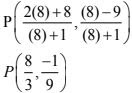# Chapter 7 Coordinate Geometry NCERT Exemplar Solutions Exercise 7.3 Class 10 Maths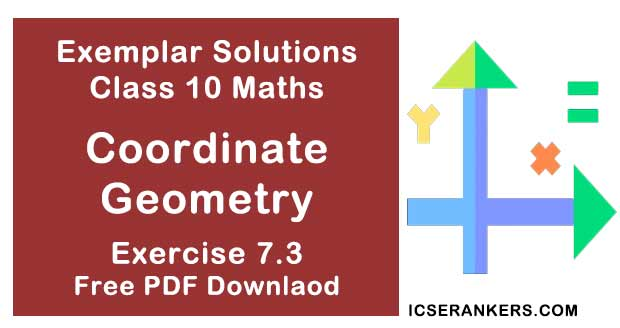Chapter Name NCERT Maths Exemplar Solutions for Chapter 7 Coordinate Geometry Exercise 7.3 Book Name NCERT Exemplar for Class 10 Maths Other Exercises Exercise 7.1Exercise 7.2Exercise 7.4 Related Study NCERT Solutions for Class 10 Maths

### Exercise 7.3 Solutions

1. Name the type of triangle formed by the points A (-5, 6), B(-4, -2) and C(7, 5)

Solution

Given points are :
A (–5, 6), B (–4, –2) and C (7, 5)
Using distance formula,
d = √[(x2 – x1)2 + (y2 – y1)2 ]
AB = √[(-4 + 5)2 + (-2 – 6)2 ]
= √[1 + 64 = √65
BC = √[(7 + 4)2 + (5 + 2)2 ]
= √(121 + 49)
= √170
AC = √[(7 + 5)2 + (5 - 6)2 ]
= √(144 + 1)
= √145
As all sides are of different length, ABC is a scalene triangle.

2. Find the points on the x–axis which are at a distance of 2√5 from the point (7, –4). How many such points are there?

Solution

Let coordinates of the point = (x, 0) (as the point lies on x axis)
x1 = 7
y1 = -4
x2 = x
y2 = 0
Distance  = √[(x2 – x1)2 + (y2 – y1)2 ]
As given in the question,
2√5 = √[(x – 7)2 + {0 – (-4)}2 ]
Squaring L.H.S and R.H.S
20 = x2 + 49 - 14x + 16
⇒ 20 = x2 + 65 - 14x
⇒ 0 = x2 - 14x + 45
⇒ 0 = x2 - 9x - 5x + 45
⇒ 0 = x(x - 9) - 5(x - 9)
⇒ 0 = (x - 9)(x - 5)
So,
x - 9 = 0
and
x - 5 = 0
Therefore,
x = 9 or x = 5
So, coordinates of points are (9, 0) or (5, 0).

3. What type of a quadrilateral do the points A (2, –2), B (7, 3), C (11, –1) and D (6, –6) taken in that order, form?

Solution

The points are A(2, -2), B(7, 3), C(11, -1) and D(6, -6)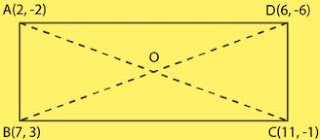Using distance formula,
d = √[(x2 – x1)2 + (y2 – y1)2 ]
AB = 5√2
BC = 4√2
CD = 5√2
DA = 4√2
Finding diagonals AC and BD, we get,
AC = √82
BD = √82
So, it is a rectangle.

4. Find the value of a, if the distance between the points A(-3, -14) and B(a, -5) is 9 units.

Solution

Distance between two points (x1 , y1) ((x2 , y2) is :
d = √[(x2 – x1)2 + (y2 – y1)2 ]
Distance between A(-3, -14) and B(a, -5) = √[(a + 3)2 + (-5 + 14)2 ] = 9
Squaring on L.H.S and R.H.S.
(a + 3)2 + 81 = 81
⇒ (a + 3)2 = 0
⇒ (a + 3)(a + 3) = 0
⇒ a + 3 = 0
⇒ a = -3

5. Find a point which is equidistant from the points A (–5, 4) and B (–1, 6)? How many such points are there?

Solution

Taking the point be P.
As given in the question,
P is equidistant from A(-5, 4) and B(-1, 6)
So,
Point P = [(x1 + x2 )/2, (y1 + y2 )/2]
= [(-5-1)/2, (6 +4)/2]
= (-3, 5)

6. Find the coordinates of the point Q on the x–axis which lies on the perpendicular bisector of the line segment joining the points A (–5, –2) and B(4, –2). Name the type of triangle formed by the points Q, A and B.

Solution

Point Q is the midpoint of AB as the point P lies on the perpendicular bisector of AB.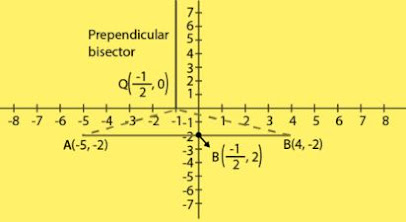By mid - point formula :
(x1 + x2 )/2 = (-5 + 4)/2
= -1/2
x = -1/2
It is given that, P lies on x axis,
y = 0
P(x, y) = (-1/2, 0)
Therefore, It is an isosceles triangle.

7. Find the value of m if the points (5, 1), (–2, –3) and (8, 2m) are collinear.

Solution

The points A(5, 1), B(-2, -3) and C(8, 2m) are collinear.
Area of ΔABC = 0
½ [ x1(y2 – y3 ) + x2 (y3 – y1) + x3 (y1 – y2) ] = 0
⇒ ½ [ 5(-3 – 2m ) + (-2) (2m – 1) + 8 {1 – (-3)} ] = 0
⇒ ½ [-15 - 10m - 4m + 2 + 32] = 0
⇒ ½ [ -14m + 19] = 0
⇒ m = 19/14

8. If the point A (2, – 4) is equidistant from P (3, 8) and Q (–10, y), find the values of y. Also find distance PQ.

Solution

The points are,
A(2, -4)
P(3, 8)
Q(-10, y)
As given in the question,
PA = QA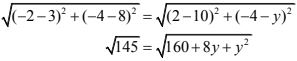Squaring both sides,
145 = 160 + 8y + y2
⇒ 160 + 8y + y2 - 145 = 0
⇒ y2 + 8y + 15 = 0
⇒ y2 + 5y + 3y + 15 = 0
⇒ y(y + 5) + 3(y + 5) = 0
⇒ (y + 5) (y + 3) = 0
⇒ y = -5
⇒ y = -3
Now,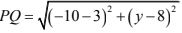For y = -3
PQ = √290
For y = -3
PQ = √338

9. Find the area of the triangle whose vertices are (-8, 4), (-6, 6) and (-3, 9).
Solution
As given in the question the vertices are:
(x1 , y1 ) = (-8, 4)
(x2 , y2 ) = (-6, 6)
(x3 , y3 ) = (-3, 4)
We have,
Area of triangle = ½ [ x1(y2 – y3 ) + x2 (y3 – y1) + x3 (y1 – y2) ]
= ½ [ -8(6 – 4) + (-6)(4 – 4) + (-3)(4 – 6) ]
= ½ [ -8(2) + (-6)(0) + (-3)(-2)]
= ½ [ -16 + 6]
= (1/2) (-10)
= -5 units

10. In what ratio does the x–axis divide the line segment joining the points (– 4, – 6) and (-1, 7) ? Find the coordinates of the point of division.
Solution
Let us take the ratio in which x - axis divides the line segment joining (-4, -6) and (-1, 7)
= 1 : k
x-coordinate = (-1 – 4k) / (k + 1)
y-coordinate = (7 – 6k) / (k + 1)
Also,
P lies on x-axis, so,
y coordinate = 0
(7 – 6k) / (k + 1) = 0
⇒ 7 – 6k = 0
⇒ k = 7/6
Hence, the ratio is
1:7/6 = 6:7
So, the coordinates of P are (-34/13, 0).

11. Find the ratio in which the point P (3/4, 5/12) divides the line segment joining the points A(1/2, 3/2) and B(2, -5).
Solution
Let P(3/4, 5/12) divide AB internally in the ratio m : n
Using the section formula,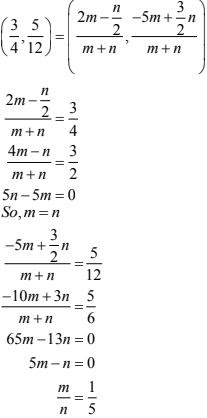So, the required ratio is 1 : 5.

12. If P (9a – 2, –b) divides line segment joining A (3a + 1, –3) and B (8a, 5) in the ratio 3 : 1, find the values of a and b.
Solution
Let us take P(9a - 2, -b) divides AB internally in the ratio 3 : 1.
By section formula,
m = 3
n = 1
So, according to question,b = -3
The required values of a and b are 1 and -3

13. If (a, b) is the mid-point of the line segment joining the points A (10, –6) and B (k, 4) and a – 2b = 18, find the value of k and the distance AB.
Solution
Since, (a, b) is the mid - point of line segment AB.
(a, b) = [(10 + k)/2, (-6+4)/2]
(a, b) = [(10+k)/2 , -1]
Equating coordinates,
a = (10 + k)/2  ...(i)
b = -1  ...(ii)
Given :
a - 2b = 18
⇒ a - 2(-1) = 18
⇒ a = 16
From equation 1.
16 = (10 +k)/2
⇒ k = 22
Therefore,
A(10, -6)
B(22, 4)
By distance formula,
AB = 2√61
The required distance of AB is 2√61.

14. The centre of a circle is (2a, a – 7). Find the values of a if the circle passes through the point (11, –9) and has diameter 10√2 units.
Solution
According to question,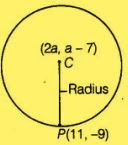distance between the centre C(2a, a - 7) and the point P(11, -9) which lie on the circle =  Radius of circle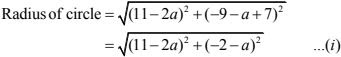Diameter = 10√2
Now from (i),
√[(11 - 2a)2 + (-2-a)2 ] = 5√2
Squaring both sides,
(11 - 2a)2 + (-2-a)2 = 50
⇒ 5a2 - 40a + 75 = 0
⇒ a2 - 8a + 15 = 0
⇒ a = 3, 5
The required values of a are 5 and 3.

15. The line segment joining the points A (3, 2) and B (5,1) is divided at the point P in the ratio 1:2 and it lies on the line 3x – 18y + k = 0. Find the value of k.
Solution
We have, the line segment joining the points 4(3,2) and 6(5,1) is divided at the point P in the ratio 1 : 2.
Coordinate of P =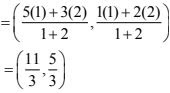Also,
Point P lies on the line 3x - 18y + k = 0,
So,
3(11/3) - 18(5/3) + k = 0
On solving,
k - 19 = 0
⇒ k = 19
The required value of k is 19.

16. If D(-1/2, 5/2), E(7, 3) and F(7/2, 7/2) are the midpoints of sides of ΔABC, find the area of the ΔABC.
Solution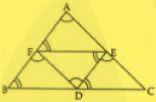In ABC triangle,
E is mid point of AC
F is mid point of AB
D is mid point of BC
As all the triangles are equal so,
ar(ABC)= 4 x ar(DEF)
Also,
D(-1/2, 5/2), E(7, 3) and F(7/2, 7/2) are the midpoints of sides of triangle ABC.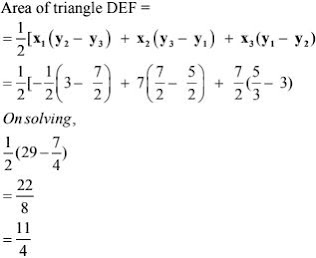So, area of ABC triangle  = 4 × (11/4)
= 11 square units
Therefore, Area of triangle ABC = 11 square units.

17. The points A (2, 9), B (a, 5) and C (5, 5) are the vertices of a triangle ABC right angled at B. Find the values of a and hence the area of ΔABC.
Solution
We have, the points A ((2, 9), B(a, 5) and C(5, 5) are the vertices of a ΔABC right angled at
B.
By Pythagoras theorem,
AC2 = AB2 + BC2 ...(i)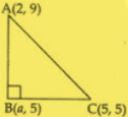AB2 = (a - 2)2 + (5 - 9)2
⇒ AB2 = a2 - 4a + 20
BC2 = (a - 5)2 + (5 - 5)2
⇒ BC2 = a2 - 10a + 25
AC2 = (5 - 2)2 + (5 - 9)2
⇒ AC2 = 25
⇒ AC = 5
Now, from (i),
5 = a2 - 4a + 20 + a2 - 10a + 25
⇒ 2a2  - 14a + 20 = 0
On solving,
a = 2, 5
Here, a cannot be equal to 5 as at a = 5, BC = 0, which is not possible.
So, a = 2
Therefore, the points becomes, A(2, 9), B(2, 5) and C(5, 5)
Area of ABC,
= ½ [ x1(y2 – y3 ) + x2 (y3 – y1) + x3 (y1 – y2) ]
= ½ [2(5 - 5) + 2(5 - 9) + 5(9 - 5)
= 6
The required area of ΔABC is 6 sq units.

18. Find the coordinates of the point R on the line segment joining the points P(-1,3) and Q(2, 5) such that PR = (3/5) PQ.
Solution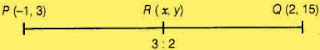Given :
PR = (3/5) PQ
⇒ PQ/PR = 5/3
⇒ (PR + RQ)/PR = 5/3
⇒ 1 + (RQ/PR) = 5/3
RQ/PR = 2/3
⇒ RQ : PR = 2 : 3
or,
PR : RQ = 3 : 2
Taking R(x, y) be the point which divides line PQ in 3:2,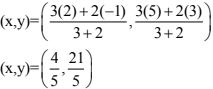19. Find the values of k if the points A (k + 1, 2k), B (3k, 2k + 3) and C (5k – 1, 5k) are collinear.
Solution
If three points are collinear then the area of triangle formed by these points is zero.
As, the points A(k + 1, 2k), B(3k, 2k + 3) and C(5k - 1, 5k) are collinear.
Then, area  of ΔABC = 0,
½ [ x1(y2 – y3 ) + x2 (y3 – y1) + x3 (y1 – y2) ]
We have,
A(k + 1, 2k)
B(3k, 2k + 3)
C(5k - 1, 5k)
So,
½ [ (k+1)(2k + 3 – 5k) + 3k(5k – 2k) + (5k - 1) (2k - 2k  + 3) ] = 0
On solving we get,
½ (6k2 - 15k + 6) = 0
⇒ 2k2 - 5k + 2 = 0
⇒ (k - 2)(2k - 1) = 0
So,
k = 2
or, k = 1/2
The required values of k are 2 and 1/2.

20. Find the ratio in which the line 2x + 3y – 5 = 0 divides the line segment joining the points (8, –9) and (2, 1). Also find the coordinates of the point of division.
Solution
Taking that the line 2x + 3y – 5 = 0 divides the line segment joining the points A (8, – 9) and B (2,1) in the ratio λ: 1 at point P.
P[(2λ + 8)/(λ+ 1)] + 3 [(λ- 9)/(λ+1)] - 5 = 0
⇒ 2(2λ + 8) + 3(λ-9) - 5(λ + 1) = 0
⇒ 2λ - 16 =0
⇒ λ = 8
So,
λ : 1 = 8 : 1
So, point P divides in the ratio, 8 : 1,